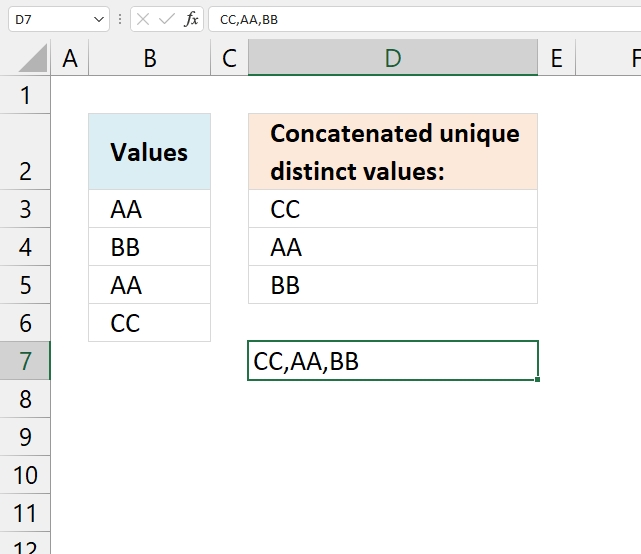Author: Oscar Cronquist Article last updated on August 09, 2021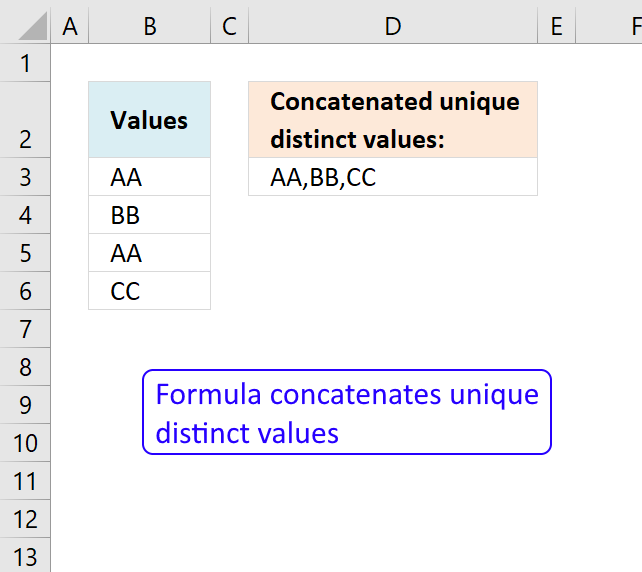This article demonstrates Excel formulas that extract unique distinct values from a cell range and concatenates the values using any delimiting characters.

Unique distinct values are all values, however, duplicates are merged into one value. For example, cell range B3:B6 contains four values. Item "AA" is displayed twice.

The formula in cell D3 returns item "AA" only once.

## 1. Concatenate unique distinct values - Excel 2019 formulaThe new TEXTJOIN function in Excel 2019 lets you do some amazing things with arrays. This post demonstrates how to extract unique distinct values from a single column and concatenate the result into one cell.

The following picture shows you 4 values in column B. Value "AA" has a duplicate in cell B5. Unique distinct values are all values except duplicates.

Array formula in cell D3:

=TEXTJOIN(", ", TRUE, IF(MATCH(B3:B6, B3:B6, 0)=MATCH(ROW(B3:B6), ROW(B3:B6)), B3:B6, ""))

Tip! You can use the UDF I made if you don't have Excel 2019 and can't use the TEXTJOIN function.

Recommended article

Concatenate cell values

Joining multiple cell values in Excel is not easy, for example, the CONCATENATE function allows you to only reference a […]

### 1.1 How to enter an array formula

1. Double press with left mouse button on cell D3
2. Copy/Paste above formula to cell D3
3. Press and hold CTRL + SHIFT
4. Press Enter once
5. Release all keys

Your formula has now a beginning and ending curly bracket, like this: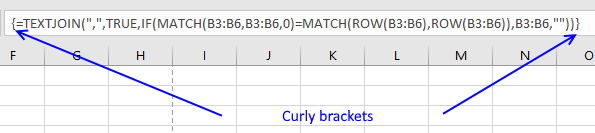Don't enter these characters yourself, they appear automatically if you followed the above steps.

Recommended article

A beginners guide to Excel array formulas

Array formulas allows you to do advanced calculations not possible with regular formulas.

### 1.2 Explaining array formula in cell D3

#### Step 1 - Match values with themselves

MATCH(B3:B6, B3:B6, 0)

becomes

MATCH({"AA"; "BB"; "AA"; "CC"}, {"AA"; "BB"; "AA"; "CC"},0)

and returns {1;2;1;4}

The MATCH function returns the relative position of a value in an array. It only returns the position of the first found value.

Value "AA" is found on the first position, so the first value in the array is 1. {1;2;1;4}

Value "BB" is found on the second position, so the second value in the array is 2. {1;2;1;4}

Value "AA" is found on the first position, so the third value in the array is 1. {1;2;1;4}

Value "CC" is found on the fourth position, so the fourth value in the array is 4. {1;2;1;4}

Recommended article

How to use the MATCH function

Identify the position of a value in an array.

#### Step 2 - Compare array with relative positions

MATCH(B3:B6, B3:B6, 0)=MATCH(ROW(B3:B6), ROW(B3:B6))

becomes

{1;2;1;4}={1;2;3;4}

and returns {TRUE;TRUE;FALSE;TRUE}

This array tells us if a value is a duplicate or not. FALSE indicates a duplicate and we don't want that in the final result.

#### Step 3 - Filter unique distinct value

IF(MATCH(B3:B6, B3:B6, 0)=MATCH(ROW(B3:B6), ROW(B3:B6)), B3:B6, "")

becomes

IF({TRUE;TRUE;FALSE;TRUE}, B3:B6, "")

becomes

IF({TRUE;TRUE;FALSE;TRUE}, {"AA";"BB";"AA";"CC"}, "")

and returns {"AA";"BB";"";"CC"}

Recommended article

How to use the IF function

Checks if a logical expression is met. Returns a specific value if TRUE and another specific value if FALSE.

#### Step 4 - Concatenate values

TEXTJOIN(", ", TRUE, IF(MATCH(B3:B6, B3:B6, 0)=MATCH(ROW(B3:B6), ROW(B3:B6)), B3:B6, ""))

becomes

TEXTJOIN(", ", TRUE, {"AA";"BB";"";"CC"})

and returns AA,BB,CC.

The first argument in the TEXTJOIN function lets you specify the delimiting character. The second argument if you want to ignore empty strings. The third argument is the values you want to concatenate.

Recommended article

How to use the TEXTJOIN function

The TEXTJOIN function is a relatively new function introduced in Excel 2019, it is like the CONCATENATE function on steroids […]

### Get the Excel fileConcatenate-unique-distinct-valuesv2.xlsx

## 2. Concatenate unique distinct values (VBA)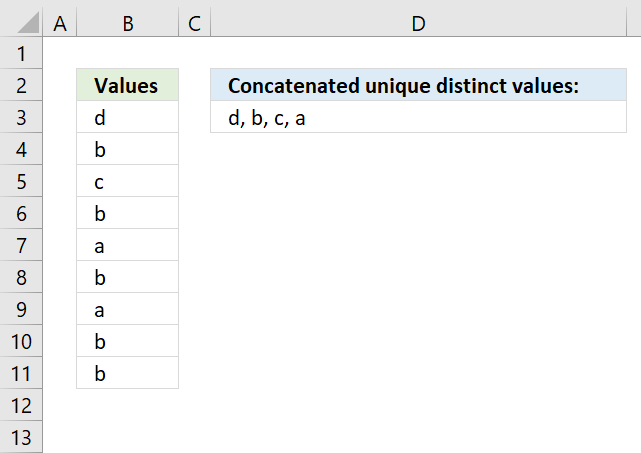The formula in cell D3 contains a User Defined Function that extracts unique distinct values concatenated, you have the option to specify the delimiting character in the second argument.

=UniqConcat(B3:B11,", ")

The formula is a regular formula, however, you need to copy the code below and paste it to a code module in your workbook before you use it.

### 2.1 VBA code

```'Name User Defined Function (UDF) and specify parameters
Function UniqConcat(rng As Range, str As String)

'Dimension variables and declare data types
Dim ucoll As New Collection, Value As Variant, temp As String

'Enable error handling, this is necessary because an error is returned when a duplicate string is added to the collection variable
On Error Resume Next

'Iterate through all values in range object rng
For Each Value In rng

'Check if number of characters in variable Value is more than 0 (zero), if so convert value to string and then add converted value to collection variable
If Len(Value) > 0 Then ucoll.Add Value, CStr(Value)

'Continue with next value
Next Value

'Disable error handling
On Error GoTo 0

'Iterate through values in collection variable ucoll
For Each Value In ucoll

'Append value to variable temp and delimiting character
temp = temp & Value & str

'Continue with next value
Next Value

'Remove last character from variable temp which is a delimiting character.
temp = Mid(temp, 1, Len(temp) - Len(str))

'Return string to cell in worksheet
UniqConcat = temp

End Function

```

### 2.2 Where to put the code?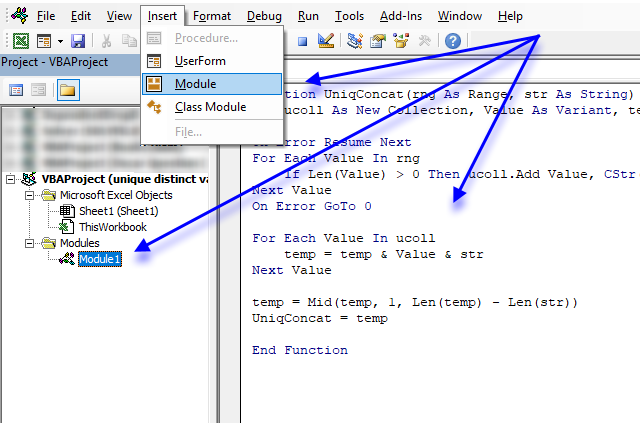1. Press Alt + F11 to open the VB Editor.
2. Press with mouse on "Insert" on the menu.
3. Press with mouse on "Module".
4. Copy above code.
5. Paste to code module.
Note, save your workbook with file extension *.xlsm (macro-enabled) in order to keep the code.

### Get the Excel fileunique-distinct-values-concatenated-to-one-cell-with-a-delimiting-character.xlsm

## 3. Concatenate unique distinct values - Excel 365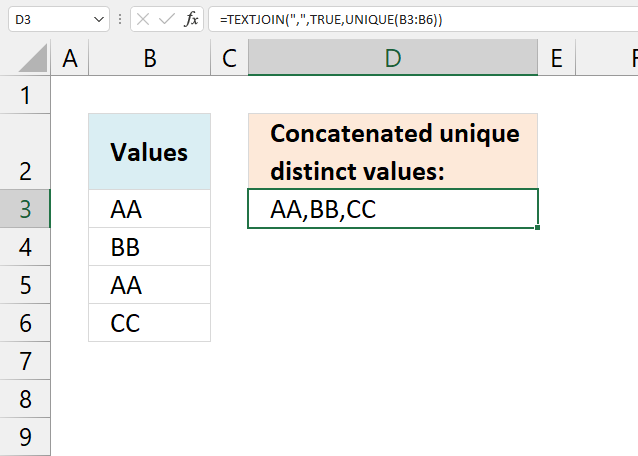Formula in cell D3:

=TEXTJOIN(",", TRUE, UNIQUE(B3:B6))

### 4.1 Explaining formula in cell D3

#### Step 1 - Extract unique distinct values

The UNIQUE function returns unique distinct values and is a new function for Excel 365 users.

UNIQUE(B3:B6)

becomes

UNIQUE({"AA";"BB";"AA";"CC"})

and returns

{"AA";"BB";"CC"}

#### Step 2 - Concatenate unique distinct values

The TEXTJOIN function allows you to combine text strings from multiple cell ranges and also use delimiting characters if you want.

TEXTJOIN(delimiterignore_emptytext1[text2], ...)

TEXTJOIN(",", TRUE, UNIQUE(B3:B6))

becomes

TEXTJOIN(",", TRUE, {"AA";"BB";"CC"})

and returns "AA,BB,CC" in cell D3.

## 4. Concatenate unique distinct values - Earlier Excel versions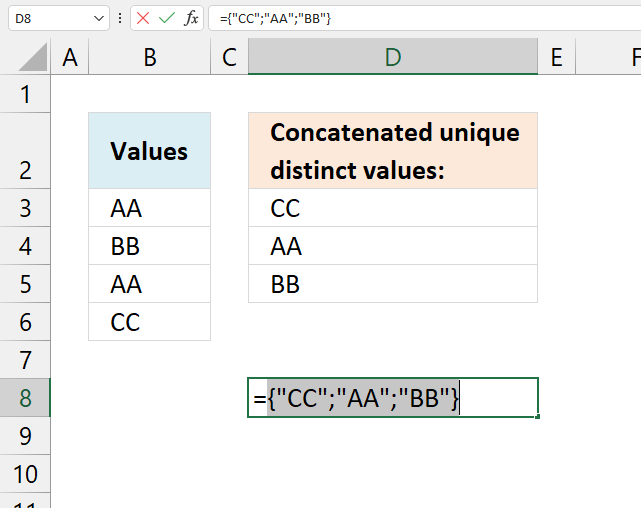Formula in cell D3:

=LOOKUP(2,1/(COUNTIF(\$D\$2:D2,\$B\$3:\$B\$6)=0),\$B\$3:\$B\$6)

This formula is explained here. Copy cell D3 and paste to cells below as far as needed.

### 4.1 Concatenate values manually

#### Step 1 - Create a cell reference to unique distinct values

Double press with left mouse button on cell D8. Press = (equal sign) and select with mouse cell range D3:D5. Alternatively, type D3:D5.

=D3:D5

Don't press Enter yet.

#### Step 2 - Convert values in cell range to array values

Press F9 on your keyboard to convert cell reference D3:D5 to actual values.

={"CC";"AA";"BB"}

#### Step 3 - Remove curly brackets and equal sign

={"CC";"AA";"BB"}

becomes

"CC";"AA";"BB"

Press Enter.

#### Step 4 - Find and Replace

Select cell D8.

Press CTRL + H to open the "Find and Replace" dialog box.

Press with the left mouse button on tab "Replace" located on the dialog box.

Type ";" in "Find what:" field, see image below.

Type , in "Replace with:" field, see the image below.

Press with left mouse button on "Replace" button.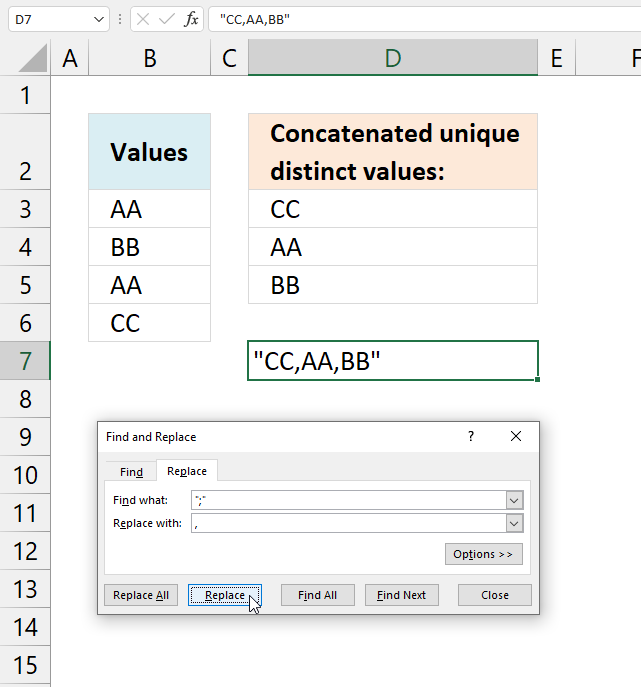Press with left mouse button on "Close" button.

#### Step 4 - Remove double quotes